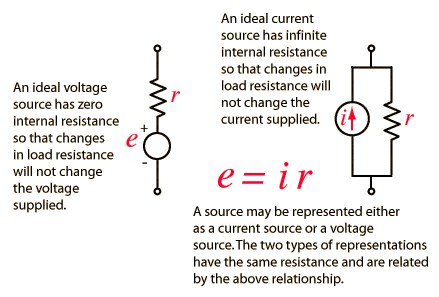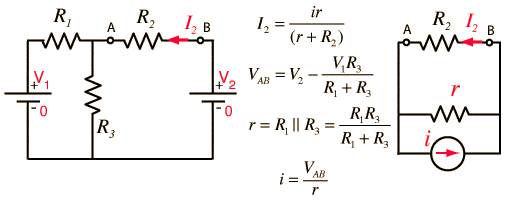# Basic electronics question - help me settle a bet

• Femme_physics

#### Femme_physics

Gold Member
I was arguing with a friend (it's been a while since she studied electronics), that you can't this turn circuit:

http://img689.imageshack.us/img689/5395/r123y.jpg [Broken]

Into the following circuit by combining R2 and R3 as though they're parallel

http://img510.imageshack.us/img510/3555/rthat.jpg [Broken]

I explained to her that as long as there's voltage source on one of the legs in parallel you can't combined them (which I believe is the correct explanation). She think you can combine the resistors R2 and R2 in that case.

So, can you help me settle this bet?

Also, and this is just something that I've wondered about: are these two circuits basically the same calculations wise?

I mean, can I combine the resistors in parallel despite the fact they're in different sides of the voltage source?

http://img510.imageshack.us/img510/1245/resisresis.jpg [Broken]

Last edited by a moderator:

You are correct R2 and R3 in the first circuit are not in parallel.
You are alos correct for the second circuit

You are correct R2 and R3 in the first circuit are not in parallel.

Can you prove why? It appears my explanation of "because there is a voltage source there" is not satisfactory.

The easiest way to think of parallel is that both ends of both devices have to be connected with no other electrical components between them (they have to have the same voltage across them).

Since the "bottom" end of the R2 is connected to a voltage source and not to the "bottom" end of R3 they are not in parallel. Even if you replaced the voltage source with another resistor R2 and R3 would not be in parallel.

There is no proof of this, the idea of series and parallel are concepts that make the math easier. Using the rule above for "parallel" you can look at a circuit and find parts that are easy to combine mathematically. But you could do away with the entire ideas of series and parallel and use something more rigorous like Kirchoff's circuit laws to solve a problem.

For the second question, yes those two circuits are the same. It doesn't matter that one resistor is drawn on one side of the voltage source from the other. You can rearrange a circuit however you want graphically as long as you do not change any connections between parts.

What you can do is convert this voltage source and that series resistor into current source and resistor in parallel.
And then you are free to do whatever you want with your resistors, because they are free of any sources directly connected to them.Last edited:
As Floid said, the ends of the resistors have to be connected, meaning they have to have the same voltage across them.

The proof is that the total current Itot = V/R1 + V/R2.
This means that the replacement resistor is:
Rtot=V/Itot
Rtot=V/(V/R1+V/R2)
Rtot=1/(1/R1+1/R2)

This does not work if there are extra components involved that make the voltage across the resistors unequal.

I explained to her that as long as there's voltage source on one of the legs in parallel you can't combined them (which I believe is the correct explanation). She think you can combine the resistors R2 and R2 in that case.

If you use Norton's theorem to work a two loop problem, you can parallel R1 and R3 in order find an equivalent resistance r as shown here:For other methods to analyze two loop d.c. circuits, follow this link.

http://hyperphysics.phy-astr.gsu.edu/hbase/electric/dcex.html#c2"

Last edited by a moderator:
There is no proof of this, the idea of series and parallel are concepts that make the math easier. Using the rule above for "parallel" you can look at a circuit and find parts that are easy to combine mathematically. But you could do away with the entire ideas of series and parallel and use something more rigorous like Kirchoff's circuit laws to solve a problem.

Thanks, that's a workable explanation IMO. And yes, Kirchhoff law does prove mathematically the fact that you can't combine them in parallel.

For the second question, yes those two circuits are the same. It doesn't matter that one resistor is drawn on one side of the voltage source from the other. You can rearrange a circuit however you want graphically as long as you do not change any connections between parts.

Hmm, interesting, but from my thinking these are NOT equal, though, right? Or are they as well?

http://img339.imageshack.us/img339/8729/noteqeqeq1.jpg [Broken]

If you use Norton's theorem to work a two loop problem, you can parallel R1 and R3 in order find an equivalent resistance r as shown here:

Thanks, but Norton theorem is beyond the scope of my studies as far as I know.

Thanks as well Bass, ILS.Last edited by a moderator:
Last edited by a moderator:
Yup.

The ends of the resistors are connected and they have the same voltage across them.

I agree with ILS. The calculated current from the battery and through the resistors will be the same whichever circuit you use.
The only (very subtle) difference is that the left hand circuit is 2 loops which produces 2 current loops. The current from the battery splits at the top and half goes to the right and half goes to the left. This means that those wires could be thinner than in the right hand circuit.
This is the principle in a domestic 'ring main' circuit so that thinner wire can be used (cheaper)

Yup.

The ends of the resistors are connected and they have the same voltage across them.

And what about combining resistors out of order? Say, in this case, instead of combining R2 and R3 first, I combine R1 and R3 first. Is that ok?

http://img268.imageshack.us/img268/5236/orderid.jpg [Broken]

I can basically pick any combination I like, yes? In thios case, I can combine R1 and R2, and leave R3 uncombined as well: it still would be ok, yes?

Last edited by a moderator:
I agree with ILS. The calculated current from the battery and through the resistors will be the same whichever circuit you use.
The only (very subtle) difference is that the left hand circuit is 2 loops which produces 2 current loops. The current from the battery splits at the top and half goes to the right and half goes to the left. This means that those wires could be thinner than in the right hand circuit.
This is the principle in a domestic 'ring main' circuit so that thinner wire can be used (cheaper)

Excellent point!

Yes.

yes... these are identical as far as doing calculations is concerned

Is this still about settling the debt?
What did you bet?

yes... these are identical as far as doing calculations is concerned

Thanks!Phew, and I'm outthat was orgasmic.

Is this still about settling the debt?
What did you bet?

No, the last parts were purely for my own knowledge. The bet was strictly for the uppermost question at the beginning of this thread (whether you combine in parallel a resistor with a voltage source there).

Believe it or not the bet says that if I win we do more physics together with my friend!(If I lost I'd have been forced to watch stupid TV shows! Thank goodness)

Yea, we do silly bets. Should've asked for hours of back massage...since I knew I was right! But still...physics...you know...I'd lose on purpose for one or the other. ;)

Yea, we do silly bets. Should've asked for hours of back massage...since I knew I was right! But still...physics...you know...This thread just got really interesting :D

This is the principle in a domestic 'ring main' circuit so that thinner wire can be used (cheaper)

Beware of this idea, multiple loops in domestic ring mains are forbidden.

bilge - i mis-read the original question. deleted

Surely you can just convert the two resistor/voltage source arms in parallel into a Thevenin equivalent circuit. In which case it could be represented as the two resistors in parallel and a calculated voltages source, Vth.

I realize this isn't exactly how your friend imagined it, but strictly speaking, the circuit diagrams you drew are equivalent as long as you aren't saying that each voltage source have the same value.

Pretty much what Bassalisk said, but no one really seemed to listen to him...

Tom

This thread seemed relevant! Need help with this, have worked it through it and don't seem to get any of the 4 answers, is it me being stupid?

#### Attachments

• circuit.pdf
164.3 KB · Views: 129
What would happen if you drew a wire across between the + terminals of the two batteries?

Does that make the problem easier?

Incidentally, you should start a new thread in the homework section for problems like this.

If you join the positive terminals of the 12 volt batteries together, then no current can flow in that wire since there is no voltage across the wire.

So, if you do that, the two 2 ohm resistors on the left are now in parallel and in series with the other 2 ohm resistor.

Two 2 ohm resistors in parallel equal what single resistor?

So you can work out the total current and hence the total power.